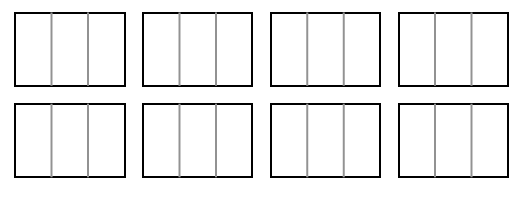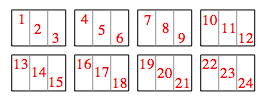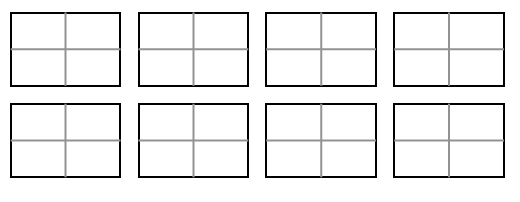Home > ACC7 > Chapter cc27 > Lesson cc27.1.3 > Problem7-35

7-35.

Study the following division problems and the diagrams that represent them. Answer the question below each diagram. Homework Help ✎

1. $8\div\frac{1}{3}$How many thirds?

$8$ wholes were each divided into $3$ sections. Now count the number of sections to get your answer.There are $24$ thirds.

1. $8\div\frac{1}{4}$How many fourths?

See part (a).